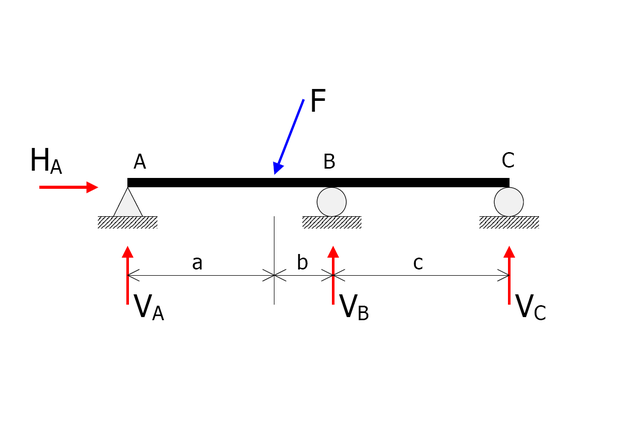What is the difference between static indeterminacy and kinematic indeterminacy ?

# What is the difference between static indeterminacy and kinematic indeterminacy ?

0
7758Statically indeterminate

In statics, a structure is statically indeterminate (or hyperstatic) when the static equilibrium equations are insufficient for determining the internal forces and reactions on that structure.Free body diagram of a statically indeterminate beam

Static Indeterminacy :-

When the number of unknown forces is more than the number of equilibrium equations required to find the forces, then the system is said to be statically indeterminate;

Let,

N1 = Number of unknown forces,

&

N2 = Number of equilibrium equations to be solved;

Then, “Q” is called the Degree of (Static) Indeterminacy,

where, “Q” is defined as follows;

Q = N1-N2